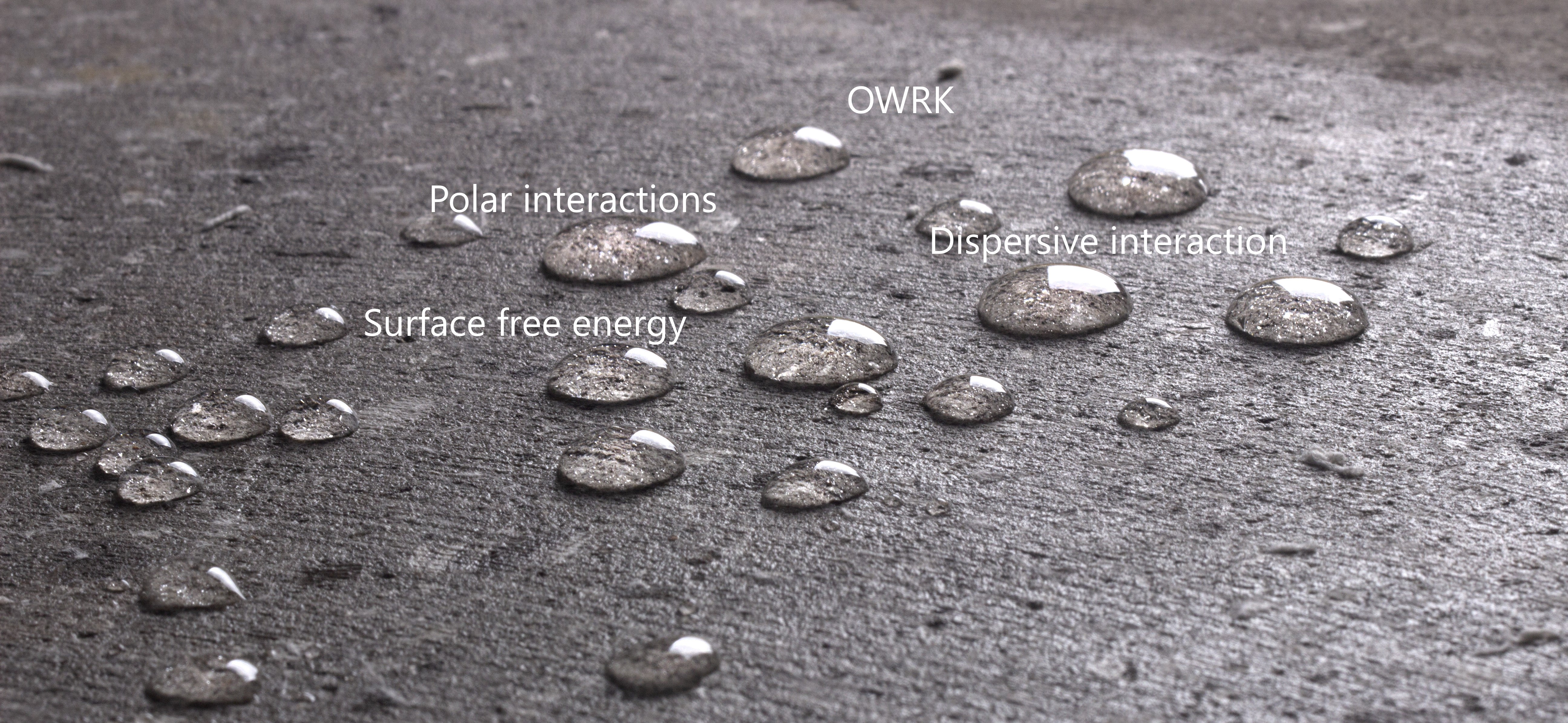Susanna Laurén Dec 22, ’20 > 5 min

# OWRK method – Owens, Wendt, Rabel and Kaelble model

## Surface Science Blog

Surface free energy cannot be measured directly, and thus several models that utilize contact angle measurements have been proposed. OWRK is one of the most used surface free energy theories. It divides the interfacial interactions into two parts: polar and dispersive. Calculation of the surface free energy with this model thus requires the measurement of the contact angle with two known liquid.

## Young’s equation is the basis of all the surface free energy theories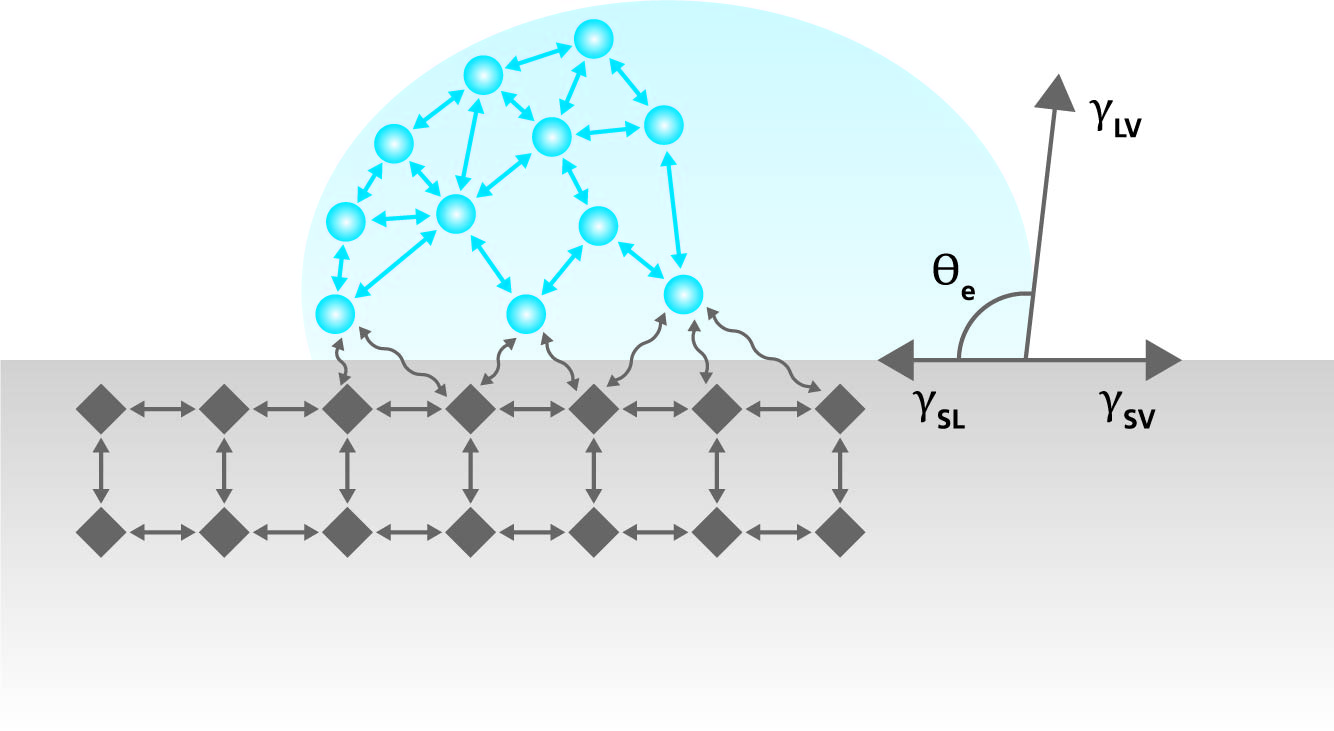Young’s equation is the basis of all the surface free energy theories. It describes the balance of forces at the three-phase contact point where air, liquid, and solid meet. Young’s equation is written as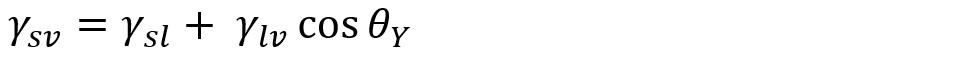,where γsv is the surface free energy of the solid, γsl is the interfacial tension between the liquid and solid, γlv is the surface tension of the liquid and the θY is the contact angle. When the surface free energy of the solid is determined the right side of the equation needs to be known or measured. Surface tension and contact angle are easy enough to measure but the interfacial tension between the liquid and the solid is more difficult.

There have been several attempts to formulate the liquid-solid interactions. Young himself, as well as Antonow, proposed thatIf γs > γl. This was later proved note to be true. Good and Girifalco formulated the equations as, where φ = 1 was assumed. This is the basis of the OWRK theory as well.

## Fowkes divided the interaction into different components

The idea of dividing the SFE into individual components includes the assumption that γsl is determined by various interfacial interactions that depend on the properties of both the measured substrate and the measurement liquid. Fowkes assumed that the surface free energy of a solid (and surface tension of a liquid) is a sum of independent components, associated with specific interactions:, where γsvd, γsvp, γsvh, γsvi and γsvab are the dispersion, polar, hydrogen, induction and acid-base components, respectively. γsvo refers to all remaining interactions. Fowkes investigated mainly systems containing the substance (solid or liquid) in which only the dispersion interactions appear. According to Fowkes, dispersion interactions relate to London interactions, arising from the electron dipole fluctuations.

## OWRK method for surface free energy calculations

Owen and Wendt continued the Fowkes idea stating that all components in the right side of the equation, except γsvd, can be considered polar (γsvp). Combining the multicomponent approach with the Good and Girifalco definition of interfacial tension, the interfacial tension based on the OWRK theory can be written as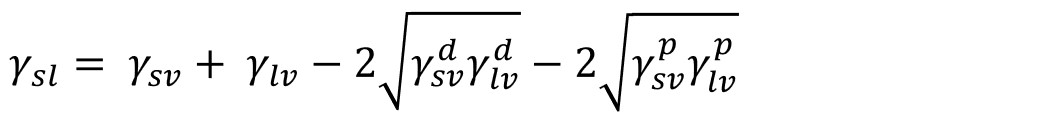And if combined with Young’s equation, the equation called OWRK can be written asBecause there are two unknowns, γsvd, and γsvp, in the equation, two liquids with the known dispersive and polar components are needed to solve it.

## Selection of the probe liquid

The liquid with the dominant polar component should be chosen as one measuring liquid and dispersive liquid as another one. This is because the interactions happen between similar components. If we would utilize only dispersive liquid, the possible polar interactions would be left unnoticed.

Water, glycerol, and formamide can be used as polar liquids and diiodomethane and α-bromonaphthalene as dispersive. Water and diiodomethane are most often utilized. Water is an obvious choice due to its large polar component, availability, and non-toxic nature. The choice of the dispersive liquid is more complex. Most of the dispersive liquids available have a very low surface tension which will lead to zero contact angles on most of the surface. Thus, more exotic probe liquids are needed. OWRK is one of the most common methods for SFE calculations.## Related products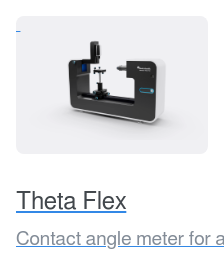## Explore the blog

You have only scratched the surface.